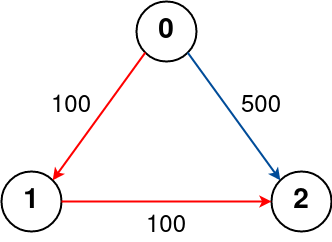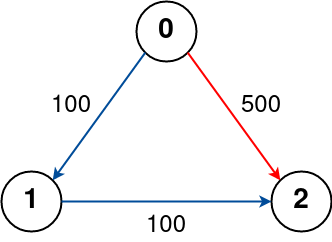## Algorithm

Problem Name: 787. Cheapest Flights Within K Stops

There are `n` cities connected by some number of flights. You are given an array `flights` where `flights[i] = [fromi, toi, pricei]` indicates that there is a flight from city `fromi` to city `toi` with cost `pricei`.

You are also given three integers `src`, `dst`, and `k`, return the cheapest price from `src` to `dst` with at most `k` stops. If there is no such route, return `-1`.

Example 1:```Input: n = 4, flights = [[0,1,100],[1,2,100],[2,0,100],[1,3,600],[2,3,200]], src = 0, dst = 3, k = 1
Output: 700
Explanation:
The graph is shown above.
The optimal path with at most 1 stop from city 0 to 3 is marked in red and has cost 100 + 600 = 700.
Note that the path through cities [0,1,2,3] is cheaper but is invalid because it uses 2 stops.
```

Example 2:```Input: n = 3, flights = [[0,1,100],[1,2,100],[0,2,500]], src = 0, dst = 2, k = 1
Output: 200
Explanation:
The graph is shown above.
The optimal path with at most 1 stop from city 0 to 2 is marked in red and has cost 100 + 100 = 200.
```

Example 3:```Input: n = 3, flights = [[0,1,100],[1,2,100],[0,2,500]], src = 0, dst = 2, k = 0
Output: 500
Explanation:
The graph is shown above.
The optimal path with no stops from city 0 to 2 is marked in red and has cost 500.
```

Constraints:

• `1 <= n <= 100`
• `0 <= flights.length <= (n * (n - 1) / 2)`
• `flights[i].length == 3`
• `0 <= fromi, toi < n`
• `fromi != toi`
• `1 <= pricei <= 104`
• There will not be any multiple flights between two cities.
• `0 <= src, dst, k < n`
• `src != dst`

## Code Examples

### #1 Code Example with C++ Programming

```Code - C++ Programming```

``````
// DFS + Brute Force
class Solution {
public:
int findCheapestPrice(int n, vector>& flights, int src, int dst, int K) {
int minPrice = INT_MAX;
vector>>g(n);
for(auto v: flights) g[v].push_back({v, v});
dfs(g, src, dst, K, 0, minPrice);
return minPrice == INT_MAX ? -1 : minPrice;
}

void dfs(vector>>& g, int cur, int dst, int K, int price, int& minPrice){
if(cur == dst){
minPrice = min(minPrice, price);
return;
}
if(K == -1 || price >= minPrice) return;
for(auto v: g[cur]) dfs(g, v, dst, K - 1, price + v, minPrice);
}
};

// BFS + Priority_queue
class Solution {
public:
int findCheapestPrice(int n, vector>& flights, int src, int dst, int K) {
vector>g(101);
vector>w(101, vector(101));
for (auto& v: flights) {
int a = v;   // src
int b = v;   // dst
int c = v;   // weight
g[a].push_back(b);
w[a][b] = c;
}
auto comp = [](vector& v1, vector& v2) {
return v1 > v2;
};
priority_queue, vector>, decltype(comp)>pq(comp);
pq.push({src, 0, K});
while (!pq.empty()) {
auto v = pq.top();
pq.pop();
int from = v;
int cost = v;
int stop = v;

if (from == dst) {
return cost;
}
if (stop < 0) {
continue;
}
--stop;
for (int x: g[from]) {
pq.push({x, cost + w[from][x], stop});
}
}
return -1;
}
};
``````
Copy The Code &

Input

cmd
n = 4, flights = [[0,1,100],[1,2,100],[2,0,100],[1,3,600],[2,3,200]], src = 0, dst = 3, k = 1

Output

cmd
700

### #2 Code Example with Javascript Programming

```Code - Javascript Programming```

``````
const findCheapestPrice = function(n, flights, src, dst, K) {
let mn = new Array(n + 1).fill(Infinity);
mn[src] = 0;
for(let k = 0; k < K + 1; k++){
let newmn = [].concat(mn);
for(let i = 0; i < flights.length; i++){
let f = flights[i], a = f, b = f, c = f;
newmn[b] = Math.min(newmn[b], mn[a] + c);
}
mn = [].concat(newmn);
}
return mn[dst] != Infinity ? mn[dst] : -1
}
``````
Copy The Code &

Input

cmd
n = 4, flights = [[0,1,100],[1,2,100],[2,0,100],[1,3,600],[2,3,200]], src = 0, dst = 3, k = 1

Output

cmd
700

### #3 Code Example with Python Programming

```Code - Python Programming```

``````
class Solution:
def findCheapestPrice(self, n, flights, src, dst, K):
flight = collections.defaultdict(list)
for s, e, p in flights:
flight[s].append((e, p))
heap = [(0, src, K + 1)]
while heap:
price, city, stop = heapq.heappop(heap)
if city == dst:
return price
elif stop > 0:
for c, p in flight[city]:
heapq.heappush(heap, (price + p, c, stop - 1))
return -1
``````
Copy The Code &

Input

cmd
n = 3, flights = [[0,1,100],[1,2,100],[0,2,500]], src = 0, dst = 2, k = 1

Output

cmd
200

### #4 Code Example with C# Programming

```Code - C# Programming```

``````
using System;

namespace LeetCode
{
public class _0787_CheapestFlightsWithinKStops
{
public int FindCheapestPrice(int n, int[][] flights, int src, int dst, int K)
{
var costs = new int[n];
for (int i = 0; i < n; i++)
costs[i] = int.MaxValue / 2;
costs[src] = 0;

for (int i = 0; i <= K; i++)
{
var currentCost = new int[n];
Array.Copy(costs, currentCost, n);

foreach (var flight in flights)
{
if (currentCost[flight] > costs[flight] + flight)
currentCost[flight] = costs[flight] + flight;
}

costs = currentCost;
}

return costs[dst] == int.MaxValue / 2 ? -1 : costs[dst];
}
}
}
``````
Copy The Code &

Input

cmd
n = 3, flights = [[0,1,100],[1,2,100],[0,2,500]], src = 0, dst = 2, k = 1

Output

cmd
200